# How do you make(draw) a horizontal line in LaTeX?

LaTeX provides us with several commands to draw horizontal lines in document.

In some cases, we need some arguments and in some cases we don’t. So in this tutorial, we will discuss all methods of drawing horizontal lines in LaTeX.

## Use \rule command with arguments for horizontal line

Easiest way to draw horizontal lines in Latex is to use the \rule[raise-height]{length}{thickness} command. And here you can pass length and thickness in arguments as you need.

You can also use an optional argument to move the line from neutral location (above or below). To increase vertical position, use a positive value and to decrease it, use a negative value.

If you want to draw a horizontal line under a paragraph, same length as the paragraph, a better option is to use \textwidth command as  length.

You can also use \noindent command to give line a better look. Below is syntax

\par\noindent\rule{\textwidth}{0.5pt}

\documentclass{article}
\usepackage{lipsum}
\begin{document}
\begin{center}
\verb|Without using \noindent|
\end{center}
\lipsum[1-3]
\par\rule{\textwidth}{0.5pt}
\par
\lipsum[1-3]
\begin{center}
\verb|Using \noindent|
\end{center}
\lipsum[1-3]
\par\noindent\rule{\textwidth}{0.5pt}
\par
\lipsum[1-3]
\end{document}

Output :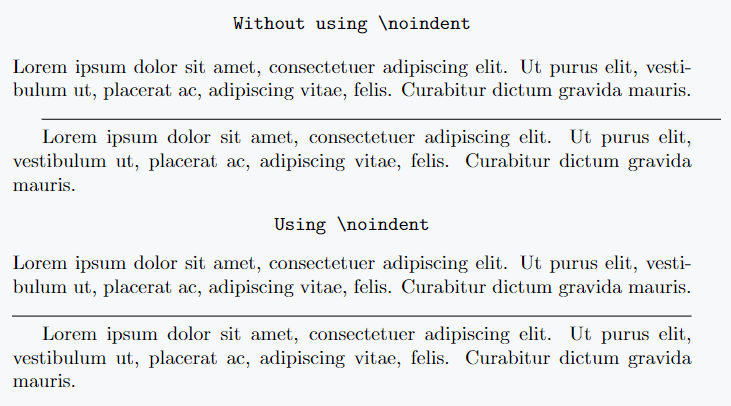## \hrule command without argument

LaTeX provides another command for drawing horizontal lines which is \hrule. This command does not require any arguments.

It is usually used between two paragraphs, so if you want some space above and below the line, you can use  \vspace{} command.

\documentclass{article}
\usepackage{lipsum}
\begin{document}
\lipsum[1-3]
\vspace{4pt}
\hrule
\vspace{4pt}
\lipsum[1-3]
\end{document}

Output :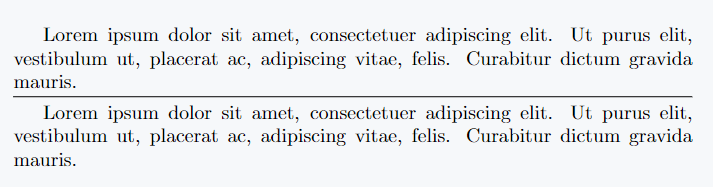## Horizontal line size is resized by \line command

Of the previous methods,  \line command is more versatile. Command allows you to set the slope for the line you want to draw. So you can draw different lines and horizontal lines too. The syntax is

\line(x-slope,y-slope){length}

For horizontal line, you need to use 1,0 as first argument and can pass length as you need. Also, to center position of line, you can use center Environment.

\documentclass{article}
\usepackage{lipsum}
\begin{document}
\lipsum[1-3]
\begin{center}
\line(1,0){340}
\end{center}
\lipsum[1-3]
\begin{center}
\line(1,0){70}
\end{center}
\end{document}

Output :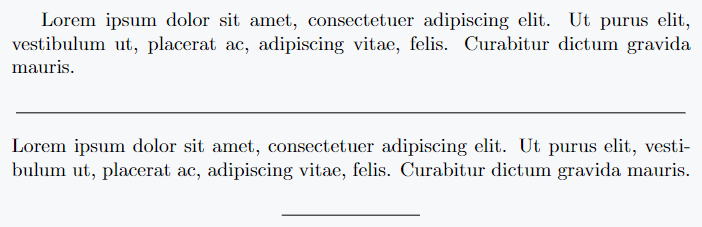## Use \hline command in LaTeX

LaTeX provides \hline command to draw horizontal lines in a simple way. This command takes no arguments.

This means you cannot customize line. For vertical space, you can use \vspace command.

You can draw double lines by using two \hline commands in a row with a small gap between lines.

\documentclass{article}
\usepackage{lipsum}
\begin{document}
\lipsum[1-3]
\vspace{5pt}
\hline
\vspace{5pt}
\lipsum[1-3]
\vspace{5pt}
\hline
\hline
\end{document}

Output :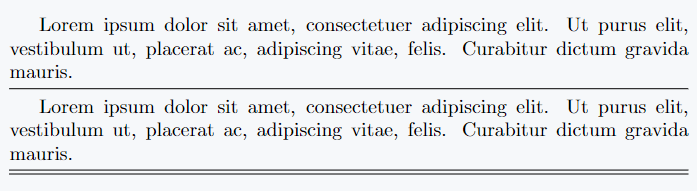## Line and text are inserted on same line by \hrulefil command

Functionality of the \hrulefill command is slightly different from above methods. Its functionality is that if there is extra space at the end of last line, then the horizontal line starts by sharing space.

You can also draw lines from next line if you want with \par command. The \hrulefill command is used by many for titles or their unique purpose.

And don’t forget to use \noindent to give the line a better look. Some examples are given below for better understanding.

\documentclass{article}
\usepackage{lipsum}
\begin{document}
\begin{center}
\verb|\hrulefill use for Title|
\end{center}
\noindent\hrulefill Title \noindent\hrulefill
\begin{center}
\verb|Without using \par|
\end{center}
\lipsum[1-2]
\noindent\hrulefill
\begin{center}
\verb|Using \par|
\end{center}
\lipsum[1-2]
\par\noindent\hrulefill
\end{document}

Output :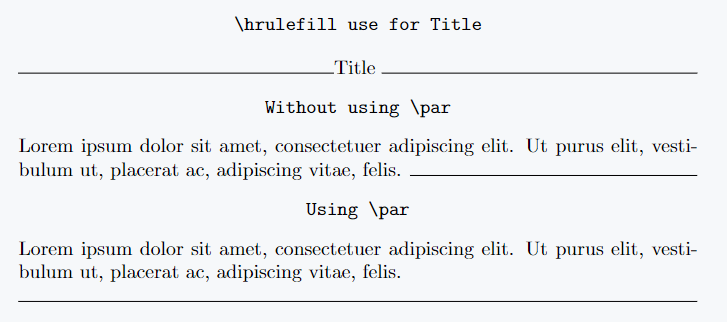## Draw horizontal dot line in LaTeX

LaTeX provides a command called \dotfill to draw horizontal dot lines. Functionality of this command is same as \hrulefill only in this case dotted line is drawn. It is generally used to give a better look to the document.

\documentclass{article}
\usepackage{lipsum}
\begin{document}
\begin{center}
\verb|\dotfill use for Title|
\end{center}
\noindent\dotfill Title \noindent\dotfill
\begin{center}
\verb|Without using \par|
\end{center}
\lipsum[1-2]
\noindent\dotfill
\begin{center}
\verb|Using \par|
\end{center}
\lipsum[1-2]
\par\noindent\dotfill
\end{document}

Output :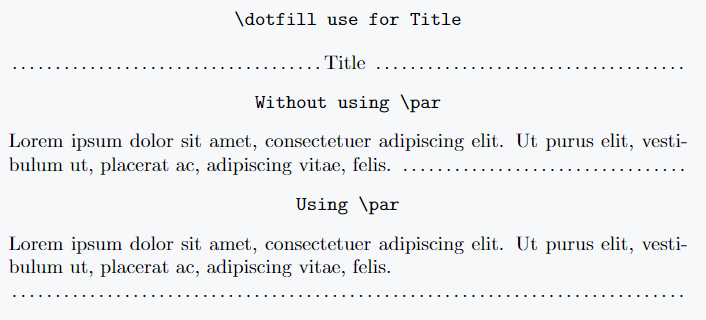#### Md Jidan Mondal

LaTeX expert with over 10 years of experience in document preparation and typesetting. Specializes in creating professional documents, reports, and presentations using LaTeX.